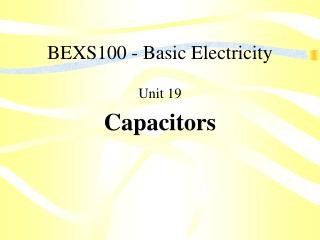Download PresentationBEXS100 - Basic ElectricityBEXS100 - Basic Electricity - PowerPoint PPT Presentation

Download PresentationBEXS100 - Basic Electricity
An Image/Link below is provided (as is) to download presentation

Download Policy: Content on the Website is provided to you AS IS for your information and personal use and may not be sold / licensed / shared on other websites without getting consent from its author. While downloading, if for some reason you are not able to download a presentation, the publisher may have deleted the file from their server.

- - - - - - - - - - - - - - - - - - - - - - - - - - - E N D - - - - - - - - - - - - - - - - - - - - - - - - - - -
Presentation Transcript

1. BEXS100 - Basic Electricity Unit 19 Capacitors

2. Unit Objectives Upon completion of this unit, you will be able to: • List the three (3) factors that determine the capacitance of a capacitor • Explain electrostatic charge • Determine the difference between polarized and nonpolarized capacitors

3. Introduction Capacitors are “devices that oppose a change of voltage”, at their base level are a simple electrical storage device yet the applications for these devices are varied and wide ranging. Capacitors can be used in timing circuits, as electronic filters, and for power factor correction among other uses. Capacitors are used in both AC and DC circuitry.

4. The Capacitance of a Capacitor The area of the plates The distance between the plates The type of “dielectric” used

5. Charging a Capacitor Electrons are removed from one plate and deposited on the opposite plate

6. Charging a Capacitor Current flows until voltage across capacitor is the same as the source voltage

7. Charging a Capacitor The capacitor remains charged after the source of voltage (battery) is removed from the circuit

8. Capacitor “Leakage” Leakage is current flow through the dielectric Proportional to the resistance of the dielectric and the charge across the plates

9. Electrostatic Charge • An “electrostatic charge” refers to electrical charges that are stationary • Static electricity is an electrostatic charge • Electrostatic charges are created by removing electrons from one plate and depositing them on the other • Energy in a capacitor is stored in the form of an electrostatic charge

10. Dielectric Stress • A lack of electrons = a positive charge • An excess of electrons = a negative charge

11. Dielectric Stress • When the atoms of the dielectric are under stress they are creating a potential energy, or stored energy • Like a drawn bow, the potential energy is being stored • When a charged capacitor is discharged it can produce currents of thousands of amperes for short periods of time

12. Dielectric Stress • Dielectric stress is proportional to the voltage difference between the plates • Excessive voltage can cause the dielectric to break down (short) and permit current flow between the plates • Capacitors have a voltage rating that should not be exceeded • Preventing excessive supply voltage to a capacitor is critical to its life span

13. Dielectric Constants • A number is assigned to different materials as an indicator of their “insulating quality” in a capacitor • This number is referred to as its dielectric constant • Changing the dielectric material of a capacitor changes its capacitance properties

14. Dielectric Constants • Assume that a capacitor uses “air” as its dielectric material and has a total capacitance of 1 mF • Changing the dielectric material to “dry paper” would change the capacitance of the capacitor to 3.5 mF

15. Capacitor Ratings • A “farad” is the basic unit of capacitance named after Michael Faraday, a famous scientist • Symbolized by the letter “F” • One farad = the change of 1 volt across the plates results in the movement of 1 coulomb of current • A farad is such a large basic unit of capacitance that it is seldom used for capacitor values

16. Capacitor Ratings More common designations of capacitance: • “mF” Microfarad = 1/1,000,000 • “nF” Nanofarad = 1/1,000,000,000 • “pF” Picofarad = 1/1,000,000,000,000 • The Picofarad is sometimes referred to as a micro/microfarad with the symbol “mmF”

17. Capacitors in Parallel • Has the same effect of increasing the plate area of one capacitor

18. Capacitors in Series • Has the effect of increasing the distance between the plates / reducing the total capacitance of the circuit

19. RC Time Constants Capacitors charge at an exponential rate (63.2%)

20. RC Time Constants Capacitors discharge at an exponential rate (63.2%)

21. RC Time Constants Accurately determining the RC time constant for a given capacitor in a circuit How long will it take to charge a 50 mF capacitor in a circuit with a 100,000 ohm resistor? Answer: 25 Seconds

22. The Two Basic Groups of Capacitors Polarized & Non-Polarized

23. Non-Polarized Capacitors • Not sensitive to polarity connection • May also be referred to as AC capacitors • Can be used in AC/DC circuits

24. Paper / Oil Filled Capacitors

25. Polarized Capacitors • Also known as electrolytic capacitors • They are sensitive to polarity • Used only in DC circuits • Reversing polarity could cause capacitor damage or explosion • Electrolytic capacitors can have very high capacitance in a small case

26. Variable Capacitors • Capacitance range can be changed within a certain range • Contains a moveable set of plates • The plates are interleaved with the stationary plates to increase or decrease the capacitance value

27. A Trimmer Capacitor • Contains one stationary plate and one moveable plate • An adjusting screw moves the moveable plate to change the capacitance value

28. Elizabethtown Technical CollegeBasic Electricity BEXS100/101Text: Delmar’s Standard Textbook of ElectricityUnit 19 - Capacitors